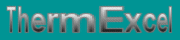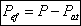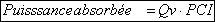Units of volume and pressure in gas industry, calorific value, heat value, gas and liquefied oil, useful output, etc.
French site
| Home | Thematic | Tables | Programs | Library | Download | Links | Contact | Forum |--

Fuel gas
 Thematic Quotation Margin Hydraulic Linear pressure loss Local pressure loss Compressible fluids No circular duct Thermal Thermal fluid flow Pipe sizing steam Control valves Orifice plate Pumps Ventilators Gas Fuel gasUnit of volume used in gas industry

The volume of reference or the cubic meter normal is often characterized by the abbreviation m³(n) or (Nm3).

This indication means that the cubic meter is given under the conditions of temperature to 0°C, under an absolute pressure.

Specific Gravity

The density of gas, relative to air, is called specific gravity. The specific gravity of air is defined as 1. Since propane gas has a specific gravity of 1.5, propane-air mixtures have a specific gravity of greater than 1.

 Unit of pressure used in the gas industry

The bar and the millibar are used for the measurement of the pressures of gas distribution.

• Low pressure: up to 50 mbar included.
• Average pressure a: from 50 mbar to 0.4 bar included.
• Average pressure b: from 0.4 bar to 4 bar included.

The legal unit of measurement of the pressure, in the international system, is the PASCAL (N/m2) and the bar.

You will find in the table attached its multiples and submultiples.

Notation:With:

• Pef = effective pressure.
• P = absolute pressure.
• Pat = Atmospheric pressure.
 Calorific value of a fuel

The calorific value of a fuel is the quantity of heat produced by its combustion, at constant pressure and under the conditions known as " normal " of temperature and pressure (i.e. to 0°C and under a pressure of 1 013 mbar).

The calorific value of natural gas is expressed in kWh per cubic meter.

The combustion of a product generates, inter alia, of water to the vapor state. Certain techniques make it possible to recover the quantity of heat contained in this water of combustion by condensing it (boilers at condensation)

One thus distinguishes two calorific values:

• The lower calorific value or Net calorific value (NCV) which supposes that the products of combustion contain the water of combustion to the vapor state. The heat contained in this water is not recovered.
• The higher calorific value or Gross calorific value (GCV) which supposes that the water of combustion is entirely condensed. The heat contained in this water is recovered.

Il existe, pour la plupart des combustibles un rapport " GVC / NVC" entre ces deux coefficients :

• Natural gas: 1.111
• Butane, Propane: 1.087
• FOD: 1.075
• FOL: 1.055
• Coal: 1.052

Since most gas burning appliances cannot utilize the heat content of the water vapor, gross calorific value is of little interest. Fuel should be compared based on the net calorific value. This is especially true for natural gas, since increased hydrogen content results in high water formation during combustion.

The natural gas makes it possible to recover, by condensation, the heat contained in the fume without there being problem of corrosion. The current outputs of the boilers with condensation can reach 105 % on NCV

Thus, while reasoning in kWh NCV, one reaches outputs higher than 100 %. The reasoning in kWh GCV is thus adapted more to energy natural .

 Calorific power and useful output

The description plates of the generators gas present two, even three displayed powers.

One finds there nominal useful output nominal, input ratings and sometimes of the minimal useful output.

The difference between these values is directly related to the output of the generator.

The nominal calorific flow rate (gas flow rate) represents the maximum calorific power of the generator.

The physical definition of the output is the relationship between the power provided (useful) to the water of heating of the generator and the power absorptive by this one. One takes account of the losses inherent in the operation.This output is also called useful output.

Thus, a boiler having a calorific flow rate of 89 kW and an useful output of 75 kW will have a useful output of 89 % approximately NCV. This generator will be able to thus feed a network of heating of a maximum power of 75 kW.

If one wants in practice to refine the calculations, it is possible to calculate the calorific power and the useful output of the generators.

The absorptive power or calorific power is the product of the volumic flow rate of gas by the NCV with constant pressure of fuel expressed in quantity of heat.• Qv = Volumic flow rate of gas.
• PCI = Lower calorific value of gas.

Last update: